# Prediction Rule Ensembles

Derives prediction rule ensembles (PREs). Largely follows the procedure for deriving PREs as described in Friedman & Popescu (2008; ), with adjustments and improvements. The main function pre() derives prediction rule ensembles consisting of rules and/or linear terms for continuous, binary, count, multinomial, and multivariate continuous responses. Function gpe() derives generalized prediction ensembles, consisting of rules, hinge and linear functions of the predictor variables.

# pre: an R package for deriving prediction rule ensembles

pre is an R package for deriving prediction rule ensembles for binary, multinomial, (multivariate) continuous, count and survival outcome variables. Input variables may be numeric, ordinal and categorical. An extensive description of the implementation and functionality is provided in Fokkema (2017). The package largely implements the algorithm for deriving prediction rule ensembles as described in Friedman & Popescu (2008), with several adjustments:

1. The package is completely R based, allowing users better access to the results and more control over the parameters used for generating the prediction rule ensemble.
2. The unbiased tree induction algorithms of Hothorn, Hornik, & Zeileis (2006) is used for deriving prediction rules, by default. Alternatively, the (g)lmtree algorithm of Zeileis, Hothorn, & Hornik (2008) can be employed, or the classification and regression tree (CART) algorithm of Breiman, Friedman, Olshen, & Stone (1984).
3. The package supports a wider range of response variable types.
4. The package allows for plotting the final rule ensemble as a collection of simple decision trees.
5. The initial ensembles may be generated as in bagging, boosting and/or random forests.
6. Hinge functions of predictor variables may be included as baselearners, like in the multivariate adaptive regression splines method of Friedman (1991), using function `gpe()`.

Note that pre is under development, and much work still needs to be done. Below, a short introductory example is provided. Fokkema (2017) provides an extensive description of the fitting procedures implemented in function `pre()` and example analyses with more extensive explanations.

## Example: Predicting ozone levels

To get a first impression of how function `pre()` works, we will fit a prediction rule ensemble to predict Ozone levels using the `airquality` dataset. We fit a prediction rule ensemble using function `pre()`:

Note that the random seed was set first, to allow for later replication of the results, as the fitting procedure depends on random sampling of training observations.

We can print the resulting ensemble (alternatively, we could use the `print` method):

The cross-validated error printed here is calculated using the same data as was used for generating the rules and therefore may provide an overly optimistic estimate of future prediction error. To obtain a more realistic prediction error estimate, we will use function `cvpre()` later on.

The table represents the rules and linear terms selected for the final ensemble, with the estimated coefficients. For rules, the `description` column provides the conditions. If all conditions of a rule apply to an observation, the predicted value of the response increases by the estimated coefficient, which is printed in the `coefficient` column. If linear terms were selected for the final ensemble (which is not the case here), the winsorizing points used to reduce the influence of outliers on the estimated coefficient would be printed in the `description` column. For linear terms, the estimated coefficient in `coefficient` reflects the increase in the predicted value of the response, for a unit increase in the predictor variable.

If we want to plot the rules in the ensemble as simple decision trees, we can use the `plot` method. Here, we request the nine most important baselearners are requested here through specification of the `nterms` argument. Through the `cex` argument, we specify the size of the node and path labels: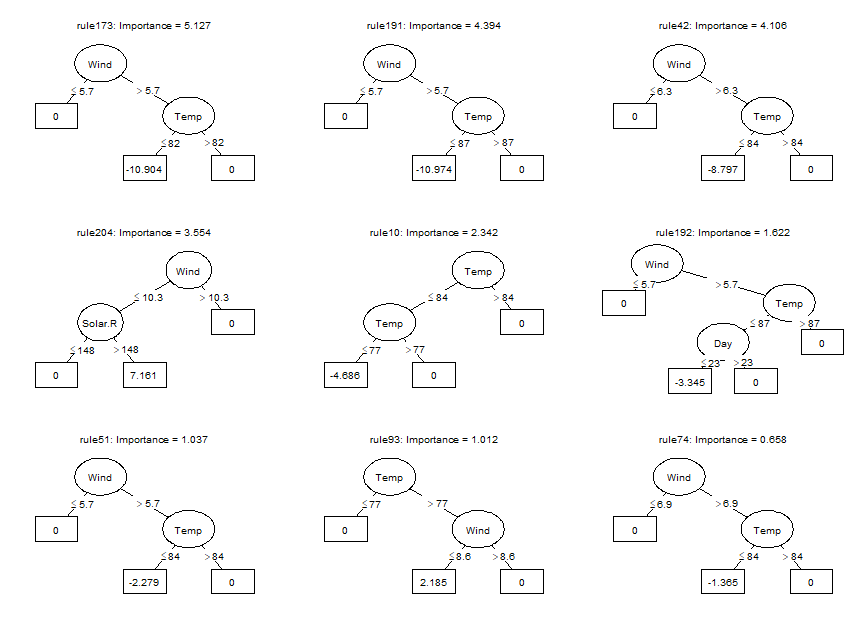We can obtain the estimated coefficients for each of the baselearners using the `coef` method (only the first ten are printed here):

We can generate predictions for new observations using the `predict` method:

We can assess the expected prediction error of the prediction rule ensemble through cross validation (10-fold, by default) using the `cvpre()` function:

The results provide the mean squared error (MSE) and mean absolute error (MAE) with their respective standard errors. The cross-validated predictions, which can be used to compute alternative estimates of predictive accuracy, are saved in `airq.cv\$cvpreds`. The folds to which observations were assigned are saved in `airq.cv\$fold_indicators`.

### Tools for interpretation

Package pre provides several additional tools for interpretation of the final ensemble. These may be especially helpful for complex ensembles containing many rules and linear terms.

We can assess the relative importance of input variables as well as baselearners using the `importance()` function: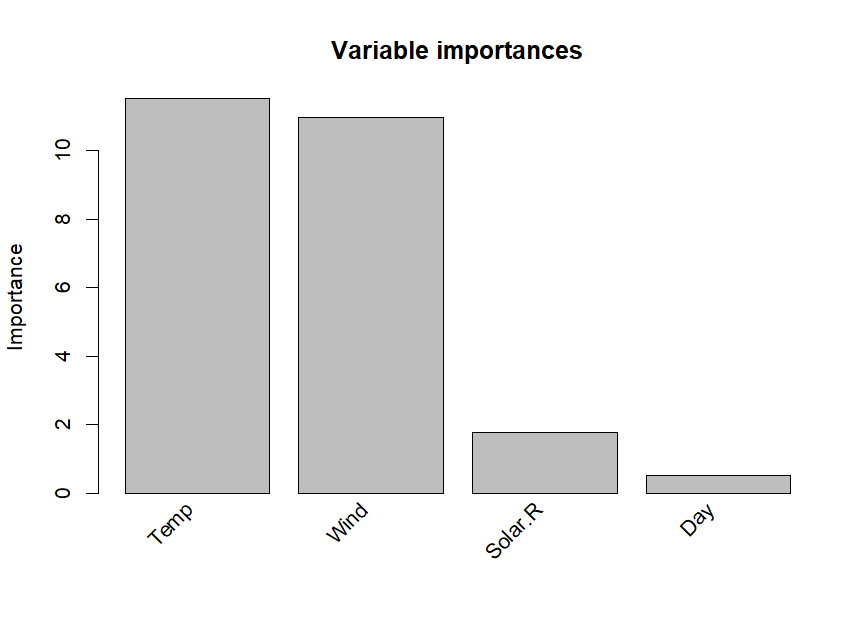As we already observed in the printed ensemble, the plotted variable importances indicate that Temperature and Wind are most strongly associated with Ozone levels. Solar.R and Day are also associated with Ozone levels, but much less strongly. Variable Month is not plotted, which means it obtained an importance of zero, indicating that it is not associated with Ozone levels. We already observed this in the printed ensemble: Month was not selected as a linear term and did not appear in any of the selected rules. The variable and baselearner importances are saved in `imps\$varimps` and `imps\$baseimps`, respectively.

We can obtain partial dependence plots to assess the effect of single predictor variables on the outcome using the `singleplot()` function: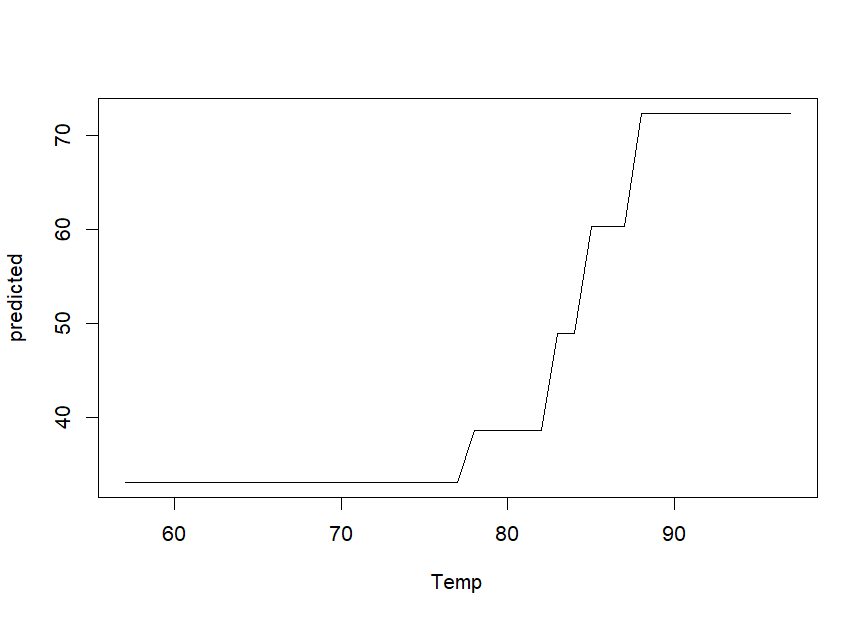We can obtain partial dependence plots to assess the effects of pairs of predictor variables on the outcome using the `pairplot()` function: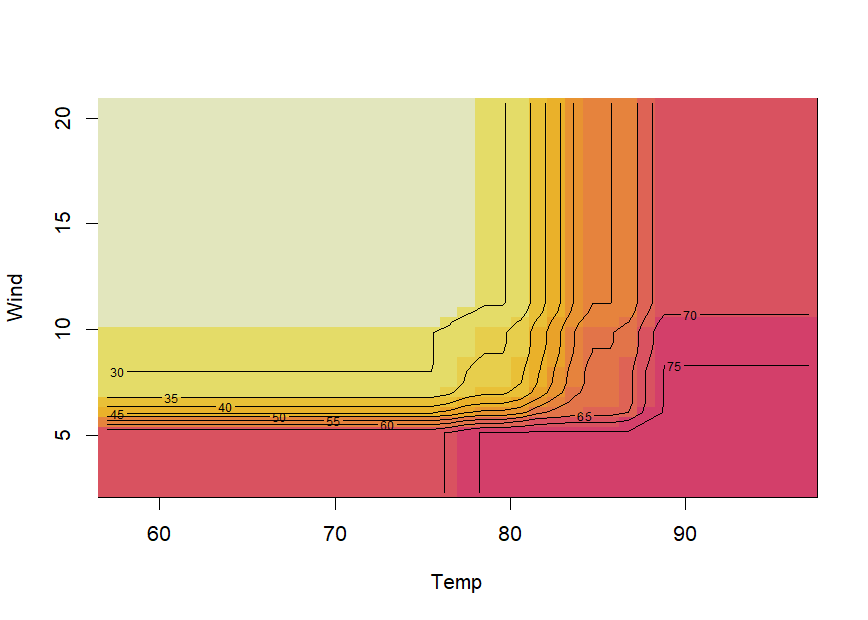Note that creating partial dependence plots is computationally intensive and computation time will increase fast with increasing numbers of observations and numbers of variables. `R` package `plotmo` created by Stephen Milborrow (2018) provides more efficient functions for plotting partial dependence, which also support `pre` models.

If the final ensemble does not contain a lot of terms, inspecting individual rules and linear terms through the `print` method may be (much) more informative than partial dependence plots. One of the main advantages of prediction rule ensembles is their interpretability: the predictive model contains only simple functions of the predictor variables (rules and linear terms), which are easy to grasp. Partial dependence plots are often much more useful for interpretation of complex models, like random forests for example.

We can obtain explanations of the predictions for individual observations using function `explain()`:The values of the rules and linear terms for each observation are saved in `expl\$predictors` and the contributions in `expl\$contribution`.

We can assess correlations between the baselearners appearing in the ensemble using the `corplot()` function: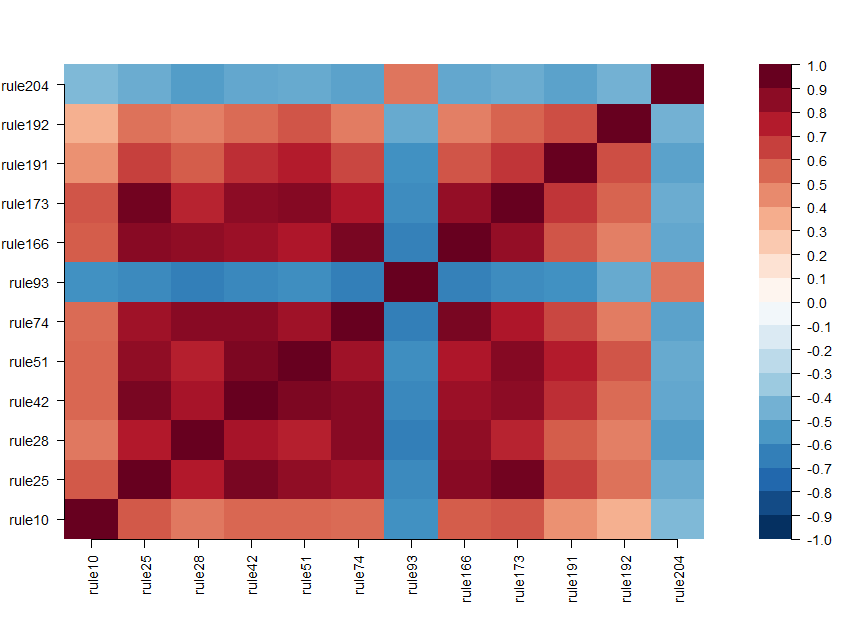### Assessing presence of interactions

We can assess the presence of interactions between the input variables using the `interact()` and `bsnullinteract()` funtions. Function `bsnullinteract()` computes null-interaction models (10, by default) based on bootstrap-sampled and permuted datasets. Function `interact()` computes interaction test statistics for each predictor variables appearing in the specified ensemble. If null-interaction models are provided through the `nullmods` argument, interaction test statistics will also be computed for the null-interaction model, providing a reference null distribution.

Note that computing null interaction models and interaction test statistics is computationally very intensive.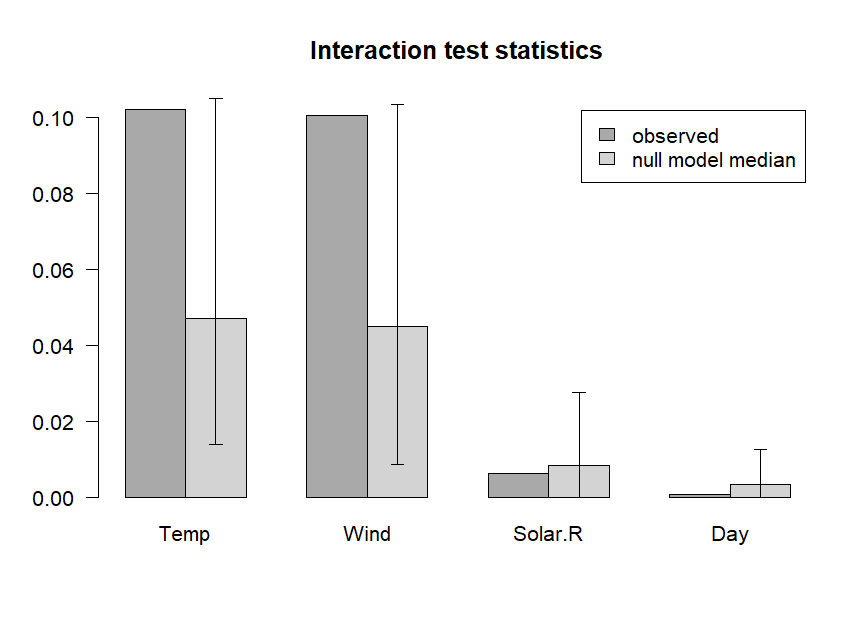The plotted variable interaction strengths indicate that Temperature and Wind may be involved in interactions, as their observed interaction strengths (darker grey) exceed the upper limit of the 90% confidence interval (CI) of interaction stengths in the null interaction models (lighter grey bar represents the median, error bars represent the 90% CIs). The plot indicates that Solar.R and Day are not involved in any interactions. Note that computation of null interaction models is computationally intensive. A more reliable result can be obtained by computing a larger number of boostrapped null interaction datasets, by setting the `nsamp` argument of function `bsnullinteract()` to a larger value (e.g., 100).

## Including hinge functions (multivariate adaptive regression splines)

More complex prediction ensembles can be obtained using the `gpe()` function. Abbreviation gpe stands for generalized prediction ensembles, which can also include hinge functions of the predictor variables as described in Friedman (1991), in addition to rules and/or linear terms. Addition of hinge functions may further improve predictive accuracy. See the following example:

## References

Breiman, L., Friedman, J., Olshen, R., & Stone, C. (1984). Classification and regression trees. Chapman&Hall/CRC.

Fokkema, M. (2017). Pre: An R package for fitting prediction rule ensembles. arXiv:1707.07149. Retrieved from https://arxiv.org/abs/1707.07149

Friedman, J. (1991). Multivariate adaptive regression splines. The Annals of Statistics, 19(1), 1–67.

Friedman, J., & Popescu, B. (2008). Predictive learning via rule ensembles. The Annals of Applied Statistics, 2(3), 916–954. Retrieved from http://www.jstor.org/stable/30245114

Hothorn, T., Hornik, K., & Zeileis, A. (2006). Unbiased recursive partitioning: A conditional inference framework. Journal of Computational and Graphical Statistics, 15(3), 651–674.

Milborrow, S. (2018). Plotmo: Plot a model’s residuals, response, and partial dependence plots. Retrieved from https://CRAN.R-project.org/package=plotmo

Zeileis, A., Hothorn, T., & Hornik, K. (2008). Model-based recursive partitioning. Journal of Computational and Graphical Statistics, 17(2), 492–514.

# News

Changes in Version 0.7.1 (2019-04-24)

• Bugs fixed in caret_pre_model: tuning of penalty.par.val argument always yielded results for "lambda.1se" only. Results are now correctly returned for "lambda.min" and "lambda.1se". caret's varImp() and predictors() not supported (perhaps temporarily), as these would always employ default penalty.par.val of "lambda.1se".

• Bugs fixed in explain().

Changes in Version 0.7 (2019-03-30)

• Added support for sparse rule matrix, which can be invoked through sparse argument in pre(). If sparse = TRUE, memory usage will be reduced and computation speed may be improved for large datasets.

• Added function explain(), which provides (graphical) explanations of the ensemble's predictions at the individual observation level.

Changes in Version 0.6 (2018-08-03)

• Added support for survival responses (i.e., family = "cox") in pre()

• Added summary methods for pre and gpe.

• Extended support to all response variable types available in pre() for functions plot(), importance() and cvpre().

• plot.pre now allows for specifying separate plotting colors for rules with positive and negative coefficients.

• coef and print methods for pre now return descriptions for the intercept (and factor variables), thanks to suggestion by Stephen Milborrow.

• Bug fix in pre(): ordered factors no longer yield error. Implemented new argument 'ordinal' in pre(), which specifies how ordered factors should be processed.

• Bug fix in cvpre(): pclass argument now processed correctly.

• Bug fix in cvpre(): previously, SDs insteas of SEs were returned for binary classification. Accurate standard errors are returned now.

• Bugs fixed in coef.pre(), print.pre(), plot.pre() and importance() when tree.unbiased = FALSE, thanks to a bug report by Stephen Milborrow.

Changes in Version 0.5 (2018-05-07)

• Function pre() now also supports multinomial and multivariate gaussian response variables.

• Function pre() now has argument 'tree.unbiased'; if set to FALSE, the CART algorithm (as implemented in package 'rpart') is employed for rule induction.

• Argument 'maxdepth' of function pre() allows for specifying varying maximum depth across trees, through specifying a vector of length ntrees, or a random number generating function. See ?maxdepth.sampler for details.

Changes in Version 0.4 (2017-08-31)

• By default, a gradient boosting approach is now taken for all response types. That is, partykit::ctree() and a learning rate of .01 is employed by default. Alternatively, glmtree() can be employed for tree induction by sprecifying use.grad = FALSE.

• The 'family' argument in pre() now takes character strings as well as glm family objects.

• Functions pairplot() and interact() now use HCL instead of highly saturated HSV colors as default plotting colors.

• Bug fixed in plot.pre: Node directions are now in accordance with rule definition.

• Bug fixed in predict.pre: No error printed when response variable is not supplied.

Changes in Version 0.3 (2017-08-03):

• Function gpe() added, which fits general prediction ensembles. By default, it fits an ensemble of rules, linear and hinge functions. Function gpe() allows for specifying custom baselearner generating functions and a custom fitting function for the final model.

• Numerous bugs fixed, yielding faster computation times and clearer plots with more customization options.

• Added support for count responses. Function pre() now has a 'family' argument, which should be set to 'poisson' for count outcomes (the 'family' argument is set automatically to 'gaussian' for numeric response variables and to 'binomial' for binary response variables (factors)).

• A gradient boosting approach for binary outcomes is applied, by default, substantially reducing computation times. This can be turned off through the 'use.grad' argument in function pre().

• The default of the 'learnrate' argument of function pre() has been changed to .01, by default. Before, it was .01 for continuous outcomes, but 0 for binary outcomes, to reduce computation time. With gradient boosting implemented, computation time is much reduced.

• Argument 'tree.control' in function pre() allows for passing arguments to partykit tree fitting functions.

• Arguments for the cv.glmnet() function are directly passed through better use of ... . Most importantly, this means that argument 'mod.sel.crit' cannot be used anymore and should be referred to as 'type.measure' (which will be directly passed to cv.glmnet). Similarly, 'thres' and 'standardize' are not explicit arguments of function pre() anymore and can now be directly passed to cv.glmnet() using ... .

• Better use of sample weights: weights specified with the 'weights' argument in pre() are now used as weights in the subsampling procedure, instead of as observation weights in the tree-fitting procedure.

• Added corplot() function, which shows the correlation between the baselearners in the ensemble.

• Function pairplot() returns a heatmap by default, a 3D or contour plot can also be requested.

• Appearance of plot resulting from interaction() improved.

Changes in Version 0.2 (2017-04-25):

• Added print() and plot() method for objects of class pre.

• Added support for using functions like factor() and log() in formula statement of function pre(). (thanks to Bill Venables for suggesting this)

• Added support for parallel computating in functions pre(), cvpre(), bsnullinteract() and interact().

• Winsorizing points used for the linear terms are reported in the description of the base learners returned by coef() and importance(). (Thanks to Rishi Sadhir for suggesting this)

• Legend included in plot for interaction test statistics.

• Fixed importance() function to allow for selecting final ensemble with different value than 'lambda.1se'.

• Cleaned up all occurrences of set.seed()

• Fixed cvpre() function: penalty.par.val argument now included

• Many minor bug fixes.

Changes in Version 0.1 (2016-12-23):

• First CRAN release.

# Reference manual

install.packages("pre")

1.0.1 by Marjolein Fokkema, 5 months ago

https://github.com/marjoleinF/pre

Report a bug at https://github.com/marjoleinF/pre/issues

Browse source code at https://github.com/cran/pre

Authors: Marjolein Fokkema [aut, cre] , Benjamin Christoffersen [aut]

Documentation:   PDF Manual